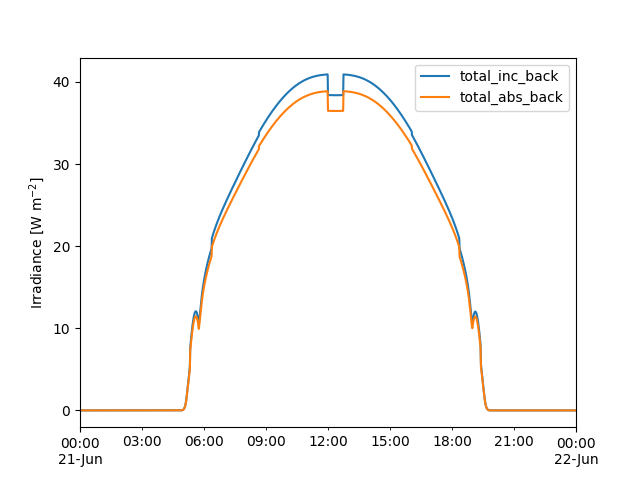# Fixed-Tilt Simulation with pvfactors#

Modeling the irradiance on the rear side of a fixed-tilt array.

Because pvfactors was originally designed for modeling single-axis tracking systems, it’s not necessarily obvious how to use it to model fixed-tilt systems correctly. This example shows how to model rear-side irradiance on a fixed-tilt array using `pvlib.bifacial.pvfactors.pvfactors_timeseries()`.

Attention

To run this example, the `solarfactors` package (an implementation of the pvfactors model) must be installed. It can be installed with either `pip install solarfactors` or `pip install pvlib[optional]`, which installs all of pvlib’s optional dependencies.

```import pandas as pd
from pvlib import location
from pvlib.bifacial.pvfactors import pvfactors_timeseries
import matplotlib.pyplot as plt
import warnings

# supressing shapely warnings that occur on import of pvfactors
warnings.filterwarnings(action='ignore', module='pvfactors')
```

First, generate the usual modeling inputs:

```times = pd.date_range('2021-06-21', '2021-06-22', freq='1T', tz='Etc/GMT+5')
loc = location.Location(latitude=40, longitude=-80, tz=times.tz)
sp = loc.get_solarposition(times)
cs = loc.get_clearsky(times)

# example array geometry
pvrow_height = 1
pvrow_width = 4
pitch = 10
gcr = pvrow_width / pitch
axis_azimuth = 180
albedo = 0.2
```

Now the trick: since pvfactors only wants to model single-axis tracking arrays, we have to pretend our fixed tilt array is a single-axis tracking array that never rotates. In that case, the “axis of rotation” is along the length of the row, with `axis_azimuth` 90 degrees offset from the fixed `surface_azimuth`.

```irrad = pvfactors_timeseries(
solar_azimuth=sp['azimuth'],
solar_zenith=sp['apparent_zenith'],
surface_azimuth=180,  # south-facing array
surface_tilt=20,
axis_azimuth=90,  # 90 degrees off from surface_azimuth.  270 is ok too
timestamps=times,
dni=cs['dni'],
dhi=cs['dhi'],
gcr=gcr,
pvrow_height=pvrow_height,
pvrow_width=pvrow_width,
albedo=albedo,
n_pvrows=3,
index_observed_pvrow=1
)

# turn into pandas DataFrame```Text(47.097222222222214, 0.5, 'Irradiance [W m\$^{-2}\$]')